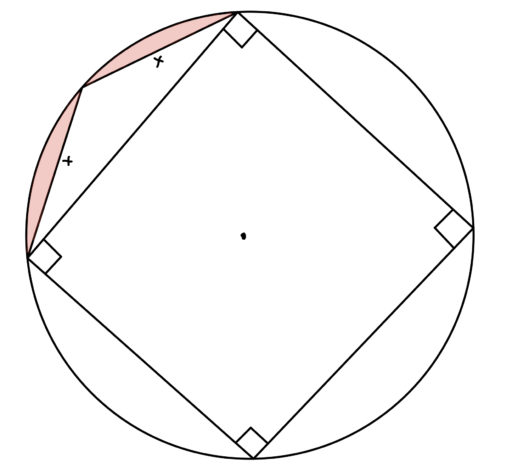Many geometry problems ask you to find the area of a shape or to compare the area of two shapes or to use the area of a shape to deduce some other value. In previous posts, we have already given the standard formulas for finding the area of such shapes (see our appendix for a full list of such formulas). But the GRE will often give you the relevant information in subtle ways, or ask you to do surprising things with the information they’ve given. So in what follows, we give some practice problems intended to help you become used to using these standard formulas in less standard ways.

But first, here are some general steps to follow in trying to solve a geometry problem:

1. Record what the problem tells you.

- The problem may give you certain lengths or angle measurements or areas or perimeters; keep track of this. It is best to write it down somewhere, either on a diagram or just in a list.

2. If you’re stuck, write down some formulas you think may be relevant.

- Thus, if the problem asks you to find the area of some shape, write down the area formula for that shape! Or if the problem gives you a 45-45-90 right triangle, write down the ratio of the side lengths. Sometimes writing such stuff down can help spark connections in your mind.

3. If you’re stuck, look back carefully at the problem and see if there’s any information you missed.

- Generally, these problems are quite parsimonious: they give you exactly what is needed to solve the problem, and no more. So if you find yourself with some unused fact, try and fit it into the problem somewhere. It’s unlikely they would’ve included something totally irrelevant to your problem.

4. If you don’t think you can get the solution quickly, just move on!

- Remember that the math section gives you 35 minutes for 20 questions, so you can only afford to spend an average of one minute and 45 seconds per question. There’s no shame in flagging a question that looks tough so that you can come back to it if time permits. Look at it this way: if you don’t get through the test, there may be easy questions down the road that you’re effectively giving up on. By flagging it and coming back later, you help ensure that you get all the low-hanging fruit in the test.

Practice Problems

1. In the following diagram,andare both squares with side lengths of 2 and area ofarea ofarea ofFind the area of the shaded rhombus.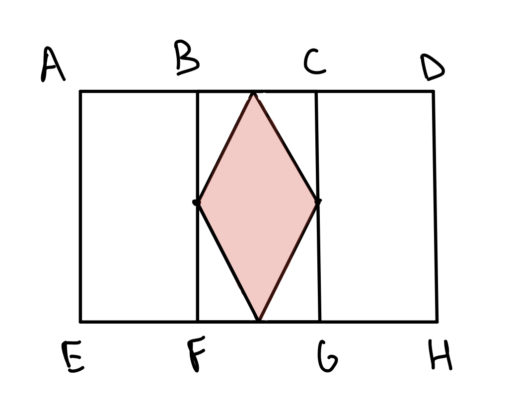2. In the following diagram,, andare equally spaced. How many scalene triangles can be formed with vertices at those points?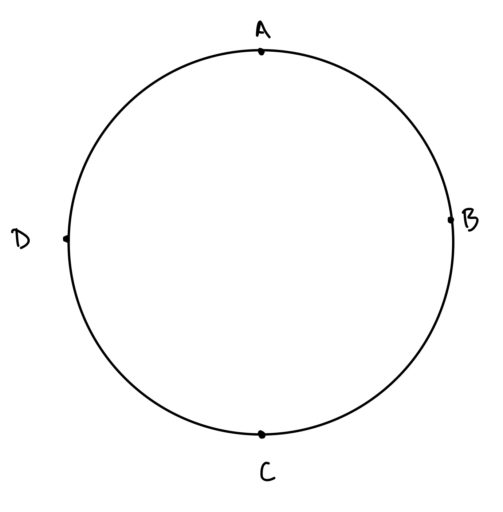3. The following points are equally spaced. How many equilateral triangles can be formed? If three distinct points are randomly chosen, what is the likelihood that they form an equilateral triangle?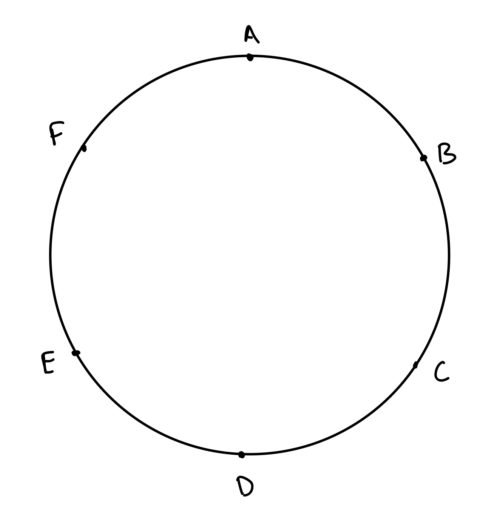4. The outer rectangle below isunits apart from the smaller rectangle on the top and bottom sides, andunit apart on the left and right sides. The inner rectangle has a height of 4 units and a base of 2 units. What is the area of the outer rectangle?5. The radius of both circles below is 1. What is the area of triangle?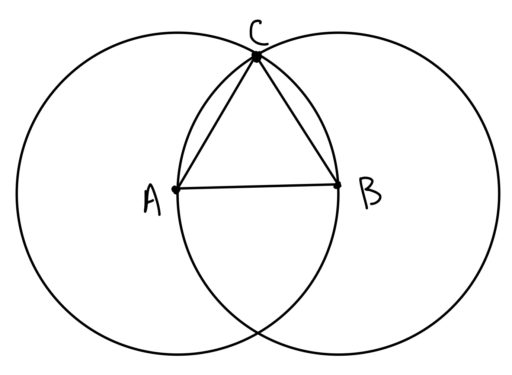6. What is the area of?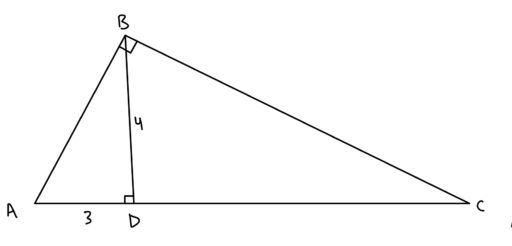7. In the following diagram,What is the area of?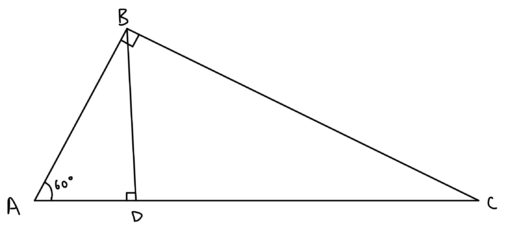Challenge Problem: You are not likely to see anything as convoluted as the following on the GRE. Still, it might be a good way to push your understanding of the above material:

8. The radius of the following circle is 5. Find the shaded area.